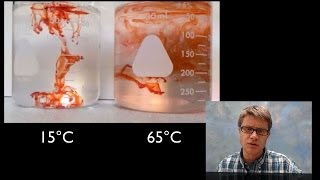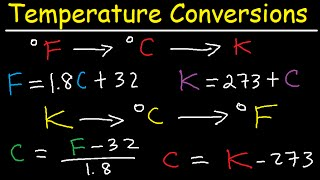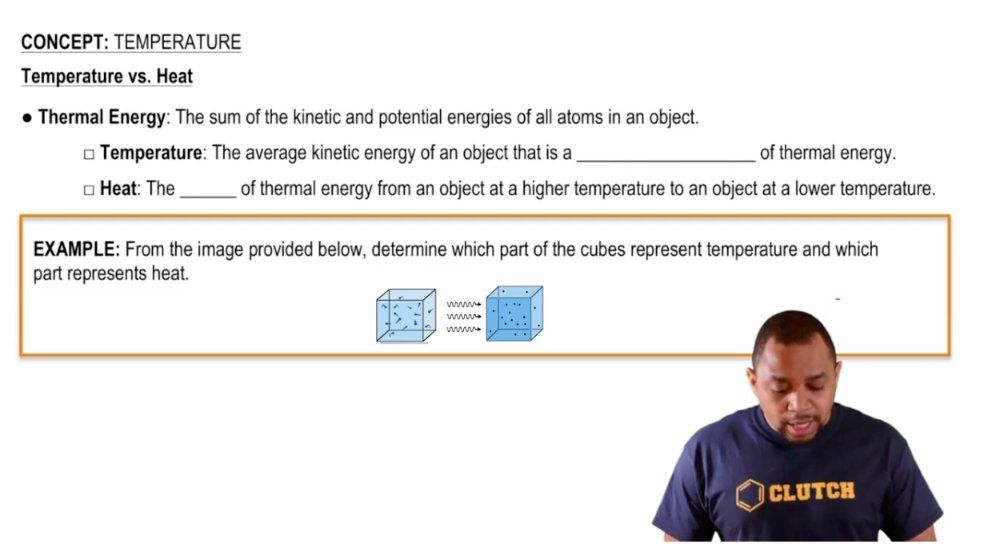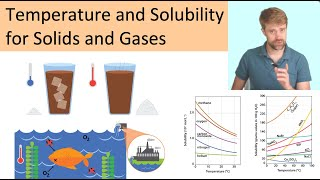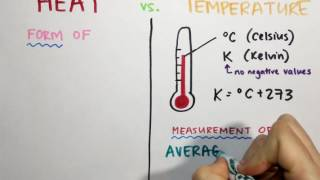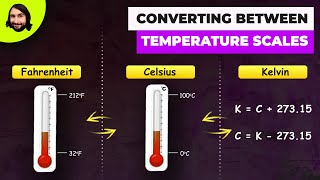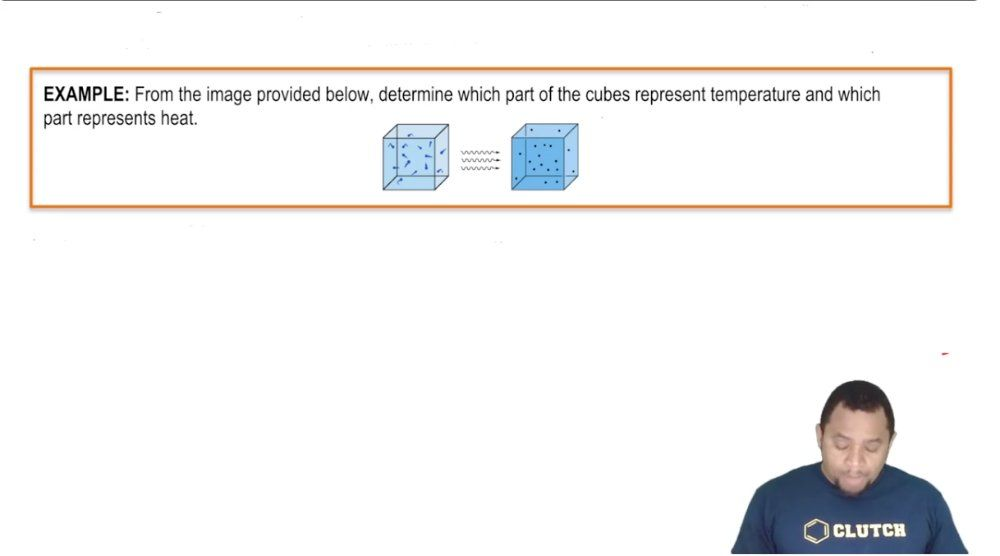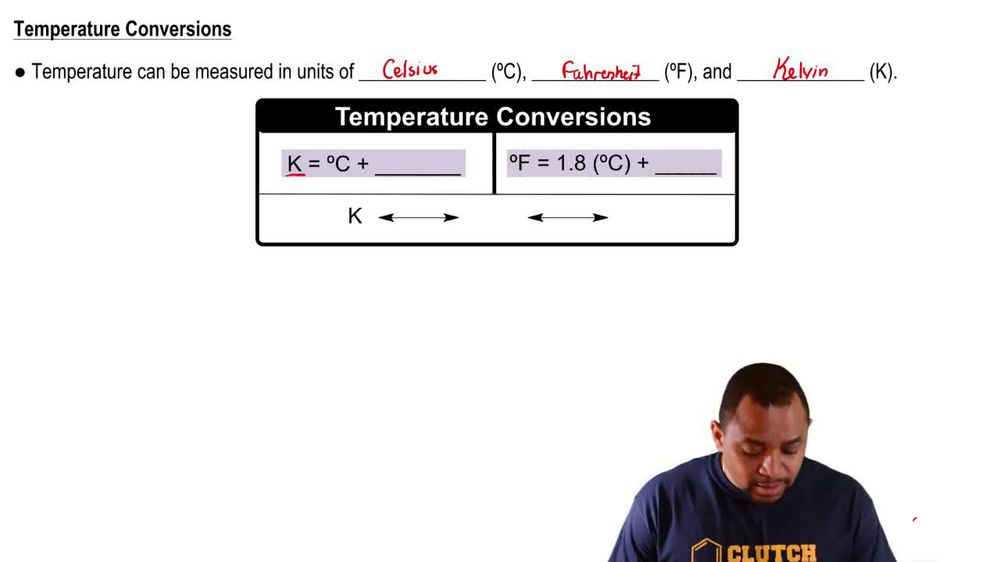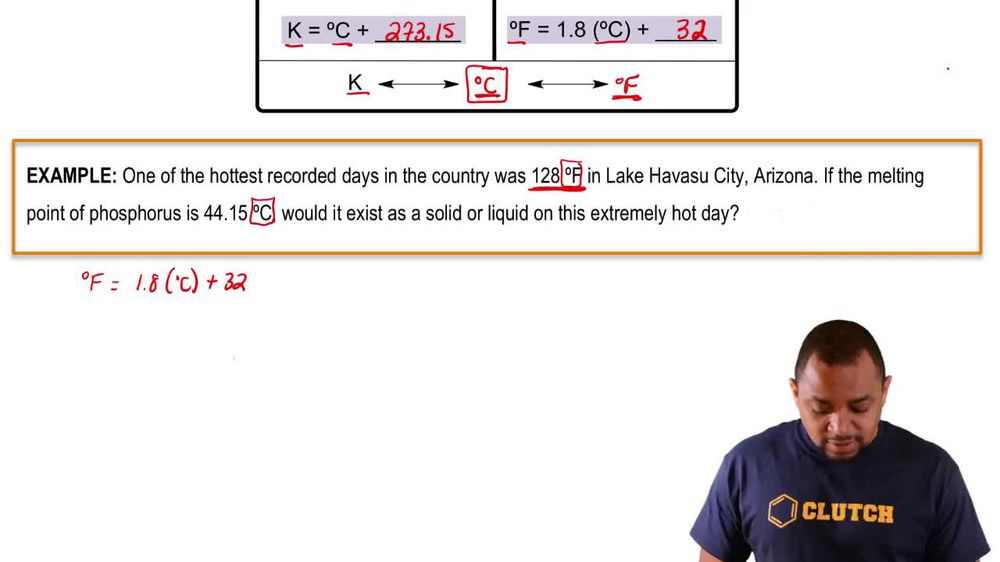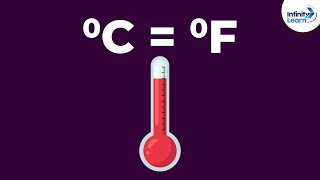Start typing, then use the up and down arrows to select an option from the list.
1. 1. Intro to General Chemistry2. Temperature
Problem

# A 125 mL sample of water at 293.2 K was heated for 8 min, 25 s so as to give a constant temperature increase of 3.0 °F/min. What is the final temperature of the water in degrees Celsius?

Relevant Solution1m
Play a video:
Hello everyone today. We have the following quality problem in order to achieve a continuous temperature increase of four F per minute. A 1 75 millimeter sample of pure iodine at 2 98 kelvin was huge for seven minutes and 30 seconds. What is the period in the final temperature? In degrees Celsius? So the first thing you wanna do is you want to find our final time or a total time And so that's going to be the seven minutes plus the half minute from the 30 seconds giving us 7 7.5 minutes. Our second goal is to be to convert kelvin to Fahrenheit. But first we need to convert kelvin into degrees Celsius and then degrees Celsius into Fahrenheit. So with Kelvin, we simply take our 298 Kelvin. Let me subtract that by our 273.15 to give us 24.85°C. Next we need to take the degrees Celsius and convert that into degrees Fahrenheit. And by doing we can go ahead and do 24.85 degrees Celsius, multiplied by 9/5 plus 32 to give us 76.73 degrees Fahrenheit. And so lastly we're going to go ahead And try and find our final temperature by doing 76.73°F plus The 7.5 minutes that we have Times our 4°F per minute interval that we have our units for a minute will cancel out and we'll be left with Fahrenheit. This gives us a final temperature of 106.73°F and 6.73 degrees Fahrenheit. And with that we've answered the question. I hope this helped, and until next time.